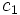# Locally conjugate conjugacy classes

This term is related to: linear representation theory
View other terms related to linear representation theory | View facts related to linear representation theory

BEWARE! This term is nonstandard and is being used locally within the wiki. [SHOW MORE]

## Definition

### Symbol-free definition

Two conjugacy classes in a group are said to be locally conjugate over a field if for any indecomposable linear representation (or equivalently, for any linear representation) over the field, the images of the conjugacy classes in the general linear group, are in the same conjugacy class of the general linear group.

### Definition with symbols

Let$G$ be a group and$k$ a field. Two conjugacy classes$c_1, c_2$ of$G$ are termed locally conjugate if for any indecomposable linear representation$\rho: G \to GL(V)$ of$G$ over$k$, and for elements$g_1 \in c_1, g_2 \in c_2$, the elements$\rho(g_1)$ and$\rho(g_2)$ are conjugate in$GL(V)$. In other words, there exists$t \in GL(V)$ such that:$t\rho(g_1)t^{-1} = \rho(g_2)$

### Definition using the L-map

Let$G$ be a group and$k$ a field. Let$C(G)$ denote the set of conjugacy classes in$G$. Let$I(G)$ denote the set of indecomposable linear representations of$G$ over$k$.

Let$L(c,\rho)$ denote the conjugacy class of$\rho(g)$ in the general linear group for any$g \in c$ (they are all in the same conjugacy class).$L$ is thus a map:$L: C(G) \times I(G) \to C(GL)$

where$C(GL)$ is the union of the set of conjugacy classes in$GL(V)$ for all vector spaces$V$ over$k$.

Then, we say that two conjugacy classes$c_1$ and$c_2$ are locally conjugate with respect to$k$ if for any$\rho \in I(G)$:$L(c_1,\rho) = L(c_2,\rho)$

## Related notions

### Locally conjugate representations

Further information: locally conjugate representations

These are representations that send each conjugacy class in the group to within the same conjugacy class in the general linear group.

### Character-conjugate conjugacy classes

Further information: character-conjugate conjugacy classes

### Class-separating field

Further information: class-separating field

A class-separating field is a field in which no two distinct conjugacy classes are locally conjugate.

## Facts

### In a sufficiently large field

A sufficiently large field for a finite group is a field which contains all the$m^{th}$ roots of unity, where$m$ is the exponent of the group. It turns out that in a sufficiently large field, no two distinct conjugacy classes are locally conjugate. This can also be rewritten as: any sufficiently large field is class-separating.

### In other fields of coprime characteristic

Take a finite group and a field whose characteristic does not divide the order of the group. Then, if the field is not sufficiently large, there may be multiple conjugacy classes that are locally conjugate to each other. In general, the following is true. Let$K$ be the smallest sufficiently large field containing the field$k$ for a finite group$G$. Then$Gal(K/k)$ includes automorphisms that raise the primitive roots of unity to some exponent. So$Gal(K/k)$ can be identified with a subgroup of the multiplicative group of the ring of integers mod$m$ where$m$ is the exponent of$G$.

Now it turns out that$c_1$ and$c_2$ are locally conjugate if and only if there exists a$r$ in this multiplicative group such that elements of$c_2$ can be expressed as$r^{th}$ powers of elements of$c_1$.

For instance:

• Suppose we take the field of real numbers and a finite group whose exponent is bigger than 2. Then the smallest sufficiently large field containing the reals is the complex numbers. The Galois group in this case is simply the subgroup of$\mathbb{Z}/m\mathbb{Z}$ comprising the elements$-1$ and$1$ (the automorphism is complex conjugation).

Thus two conjugacy classes in the group are locally conjugate over real numbers if and only if they are inverses of each other, viz one goes to the other via the inverse map.